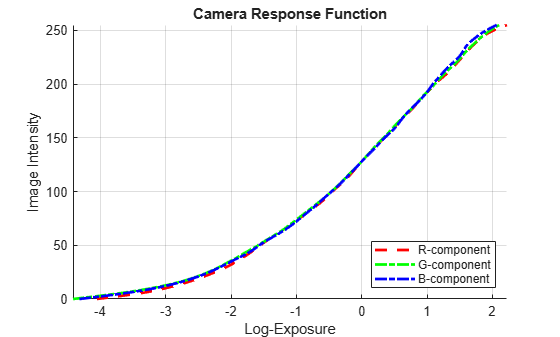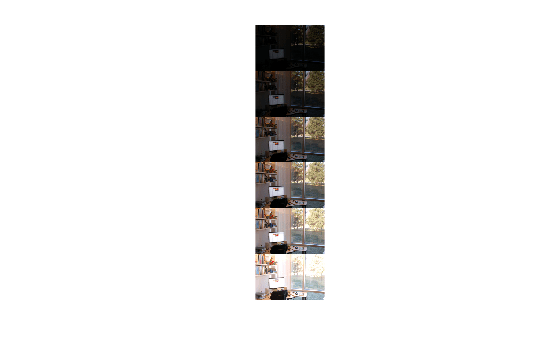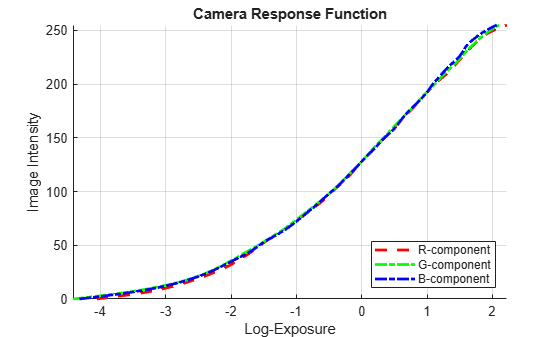# camresponse

Estimate camera response function

Since R2019a

## Syntax

``crf = camresponse(files)``
``crf = camresponse(imds)``
``crf = camresponse(___,'ExposureTimes',expTimes) ``
``crf = camresponse(images,'ExposureTimes',expTimes)``

## Description

example

````crf = camresponse(files)` estimates the camera response function from a set of spatially registered, low dynamic range (LDR) images listed in `files`.```

example

````crf = camresponse(imds)` estimates the camera response function from a set of spatially registered LDR images stored as an `ImageDatastore` object, `imds`. ```

example

````crf = camresponse(___,'ExposureTimes',expTimes) `specifies the exposure time for each image in the input set using a name-value pair. You can specify this name-value pair in addition to the input argument from any of the previous syntaxes.```
````crf = camresponse(images,'ExposureTimes',expTimes)` estimates the camera response function from a set of spatially registered LDR images stored as a cell array. Specify the exposure time for each image in the input set by using a name-value pair.```

## Examples

collapse all

Specify a set of six low dynamic range (LDR) images that are spatially registered. These LDR images have same f-stop values and varying exposure times.

```files = ["office_1.jpg","office_2.jpg","office_3.jpg",... "office_4.jpg","office_5.jpg","office_6.jpg"];```

Estimate the camera response function from the set of specified images.

`crf = camresponse(files);`

Specify the range of intensity levels in the input images.

`range = 0:length(crf)-1;`

Plot the estimated camera response function for each of the red, green, and blue color components. The plot shows the relationship between log-exposure and image intensity.

```figure hold on plot(crf(:,1),range,'--r','LineWidth',2); plot(crf(:,2),range,'-.g','LineWidth',2); plot(crf(:,3),range,'-.b','LineWidth',2); xlabel('Log-Exposure'); ylabel('Image Intensity'); title('Camera Response Function'); grid on axis('tight') legend('R-component','G-component','B-component','Location','southeast')```Create an `ImageDatastore` object containing six low dynamic range (LDR) images.

```setDir = fullfile(toolboxdir('images'),'imdata','office_*'); imds = imageDatastore(setDir);```

Display the images in a montage.

`montage(imds,'Size',[6 1])`Specify the exposure time for each image in the `ImageDatastore` object.

`expTimes = [0.0333 0.1000 0.3333 0.6250 1.3000 4.0000];`

Estimate the camera response function from the images in the datastore, specifying the exposure times.

`crf = camresponse(imds,'ExposureTimes',expTimes);`

Specify the range of intensity values in the input images.

`range = 0:length(crf)-1;`

Plot the estimated camera response function for each of the R, G, and B color components. The plot shows the relationship between log-exposure and image intensity.

```figure hold on plot(crf(:,1),range,'--r','LineWidth',2); plot(crf(:,2),range,'-.g','LineWidth',2); plot(crf(:,3),range,'-.b','LineWidth',2); xlabel('Log-Exposure'); ylabel('Image Intensity'); title('Camera Response Function'); grid on axis('tight') legend('R-component','G-component','B-component','Location','southeast')```## Input Arguments

collapse all

Set of spatially registered LDR images, specified as a string array or a cell array of character vectors. These images can be color or grayscale of any bit depth. However, the preferred bit depth for LDR images is 8 or 16.

Data Types: `char` | `string` | `cell`

Set of spatially registered LDR images, specified as an `ImageDatastore` object. These images can be color or grayscale of any bit depth. However, the preferred bit depth for LDR images is 8 or 16.

Set of spatially registered LDR images, specified as a cell array. These images can be color or grayscale of any bit depth. However, the preferred bit depth for LDR images is 8 or 16.

Exposure time of input images, specified as a numeric vector of positive values. The kth element in the vector corresponds to the kth LDR image in the input set. If you specify `expTimes`, the function overrides the EXIF exposure metadata.

Example: ```camresponse(files,'ExposureTimes',[0.1 0.3 0.4]);```

Data Types: `single` | `double`

Note

When input is a cell array of LDR images, you must specify exposure time as the second input argument by using the name-value pair `'ExposureTimes'`.

## Output Arguments

collapse all

Estimate of camera response function, returned as an n-by-1 vector for grayscale images and n-by-3 matrix for color images. The camera response function maps the log-exposure value (scene radiance) to the intensity levels in the input images. The value of n is 2bit depth. For example, if the bit depth of the input set of images is 8, then n is 256.

Data Types: `double`

## Tips

• This function requires a minimum of two images with different exposure times. A larger number of images yields a better estimate of `crf` at the expense of more processing time.

• The input image files in `files` and `imds` must contain the Exchangeable Image File Format (EXIF) exposure metadata. To estimate the `crf` values, the function reads the exposure time in the EXIF metadata. If you specify `expTimes`, the function overrides the exposure time in the EXIF metadata.

 Debevec, P.E., and J. Malik. "Recovering High Dynamic Range Radiance Maps from Photographs." In ACM SIGGRAPH 2008 classes, Article No. 31. New York, NY: ACM, 2008.